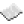# Refuel

Refuel is a built in program for turtles that will make turtles search their inventory and use any fuels they find, up to the limit specified in the command. The limit specifies the number of fuel items to be consumed, not the total amount of fuel, so "refuel 2" could consume two lava buckets (2,000 fuel total), or two wooden shovels (20 fuel total), depending on which items it first comes across in the turtle's inventory.

Usage: refuel <amount>Example Uses up to 10 fuel items Code ```refuel 10 ```

## Code

The refuel program uses the following code:

``` ```

``` local tArgs = { ... } local nLimit = 1 if #tArgs > 1 then print( "Usage: refuel [number]" ) return elseif #tArgs > 0 then if tArgs == "all" then nLimit = 64 * 16 else nLimit = tonumber( tArgs ) end end if turtle.getFuelLevel() ~= "unlimited" then for n=1,16 do local nCount = turtle.getItemCount(n) if nLimit > 0 and nCount > 0 and turtle.getFuelLevel() < turtle.getFuelLimit() then local nBurn = math.min( nLimit, nCount ) turtle.select( n ) if turtle.refuel( nBurn ) then local nNewCount = turtle.getItemCount(n) nLimit = nLimit - (nCount - nNewCount) end end end print( "Fuel level is "..turtle.getFuelLevel() ) if turtle.getFuelLevel() == turtle.getFuelLimit() then print( "Fuel limit reached" ) end else print( "Fuel level is unlimited" ) end ```